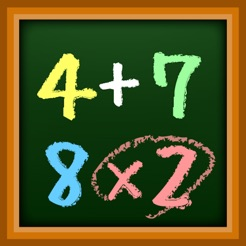Screenshots

•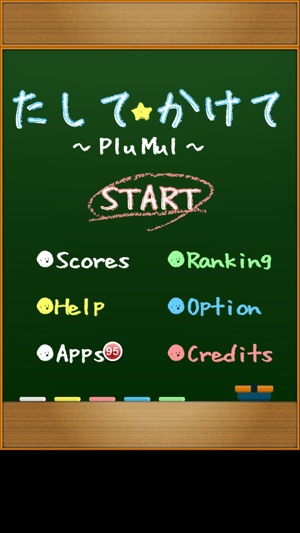•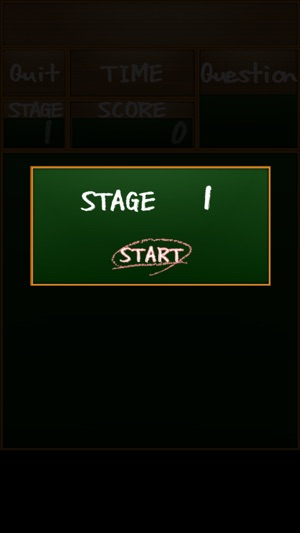•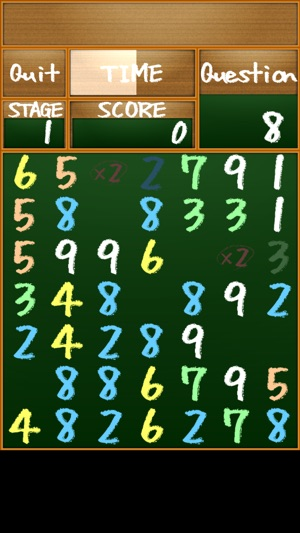•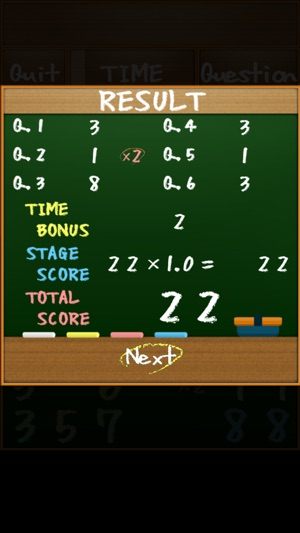•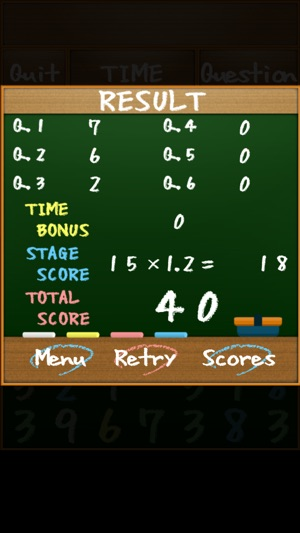Description

Add and multiply to make the same as a specific number.
Can you work out as calculated?

[How to play]
Tap arranged numbers to add. Make the same as the number in the question box.
Any calculation formulas are OK to make the same as the specific number. Add up!
Ex. 6 = 6
= 2 + 4
= 2 + 2 + 2

Just adding is not fun. Press [x2] to multiply!
Tap [x2] to double the numbers you choose.
Ex. 10 = (3 + 2) [x2]

When you make a wrong choice
Tap a chosen number again to cancel.
If the answer of your calculation formula is higher than the number in the question box, your math formula will be reset. Choose numbers again!

[Level up & Game over]
It’s correct that the answer of your calculation formula becomes the same as the number in the question box. Go on to the next question.
If you give correct answers for six consecutive times, you will go to the next stage.
The arrangement of numbers changes every stage.
The more stages you pass, the shorter allotted time you have.
When limited time runs out, it’s game over.

[The key to get a high score]
The more numbers you use, the more points you get!
Get one point per a number.
Use numbers as many as possible to increase points.
Ex. 7= 7 ⇒ 1 point
3 + 4 ⇒ 2 points
2 + 2 + 3 ⇒ 3 points

Use [x2] effectively to increase points!
A math formula including [x2] doubles points.

Ex. 12 = 3 + 3 + 6 ⇒ 3 points
= 3 x 2 + 6 ⇒ 4 points
= (2 + 1 + 3) x 2 ⇒ 6 points

[Ranking]
Press [SCORES] to check your score.
Add and multiply as much as possible to get a high ranking!

Version 1.04

- Fixed Bugs.

1.0 out of 5
1 Rating

1 Rating

Information

Seller
Yuko Hara
Size
10.3 MB
Category
Games
Compatibility

Requires iOS 4.3 or later. Compatible with iPhone, iPad, and iPod touch.

Languages

English, Japanese

Age Rating
Rated 4+
© 2011-2014 Amuzenet Inc.
Price
Free

Supports

•